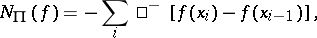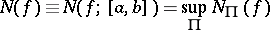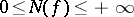# Negative variation of a function

(diff) ← Older revision | Latest revision (diff) | Newer revision → (diff)

negative increment of a function

One of the two terms whose sum is the complete increment or variation of a function on a given interval. Letbe a function of a real variable, defined on an intervaland taking finite real values.

Letbe an arbitrary partition ofand letwhere the summation is over those numbersfor which the differenceis non-positive. The quantityis called the negative variation (negative increment) of the functionon the interval. It is always true that. See also Positive variation of a function; Variation of a function.